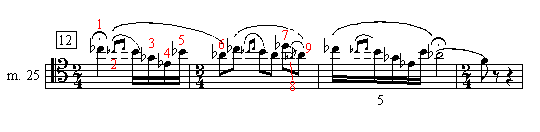Stravinsky, Igor. Rite of Spring. Entries arranged by opening solo first, then second solo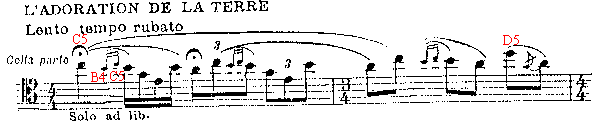Stravinsky, Igor. Rite of Spring. Corey, Gerald (25 May 1995).

```D5:
Eb
x o x | o o x Ab
d           F#```

Stravinsky, Igor. Rite of Spring (opening?). de Gomar, Juan. In Apfelstadt, Marc and Klimko, Ronald. Bassoon Performance Practice, Teaching Materials, Techniques and Methods. Idaho: University of Idaho, 1993, p. 55. Recommends fingerings for B4 and C5 found in Cooper pp. 305 and 320.

```B4:                    C5:
Eb                     Eb
x x o | x x o F        x o o | x x o F
c                      c```

Stravinsky, Igor. Rite of Spring, mm. 1-15. Moritz, Frederick. "A Dissertation on the Use of Alternate Fingerings for Some of the High Register Notes on the Heckel and Heckel- Type Bassoons." The Double Reed 8/3 (Winter 1985): 28-33. The notes are numbered in the music below. The reader is strongly encouraged to refer to the article, since the fingerings below recur throughout the solos.

In measure 1 start C5 with Cooper p. 322 (no D speaker key):

```C5. Note #1:
Eb
x o o | o o o
c```

For grace-notes B4 and C5, and sixteenth note B4:

```B4. Note #2:          C5. Note #3:
x x o | x o o F       x o o | x o o F
c                     c```

Alternate B4 fingering for the same passage and other B4-C5 grace notes in the passage (see pp. 31 and 33 of the article). No C5 fingering given.

```B4. Note #2:
Eb
x x o | x x
c```

G4 sixteenth (second beat of measure 1):

```G4.  Note # 5:
Eb
o / x | x o o F```

E4 sixteenth (second beat of m. 1):

```E4.  Note #6:
Eb
x o x | o o x```

B4 sixteenth (second beat of m. 1):

```B4.  Note #7:
Eb
x x o | x o o F
a c#```

A4 with the fermata (third beat of m. 1):

```A4. Note #8:
Eb
x x x | o o x
a c#```

A4 in measure 2, second beat:

```A4. Note #9:
Eb
/ x x | o o x Ab
c```

D5 in measure 2. Same as Cooper p. 338:

```D5. Note #10:
Eb
o o x | o o x Ab
d```

Grace-note G4 in measure 2. Optional half- hole of the L. H. D hole:

```G4. Note #11:
Eb
o x x | x o o
Bb```

A4, last eighth of measure 2. Same as Cooper p. 275:

```A4.  Note #12:
Eb
x x x | x o x
a c#  Bb```

Bb4 in measure 4 (at rehearsal number 1):

```Bb4. Note #13:
Eb
x x x | x x o
c       E```

Gb4 in measure 5 (3/4 bar). Same as Cooper p. 230:

```Gb4. Note #14:
Eb
o x x | x x o
Bb```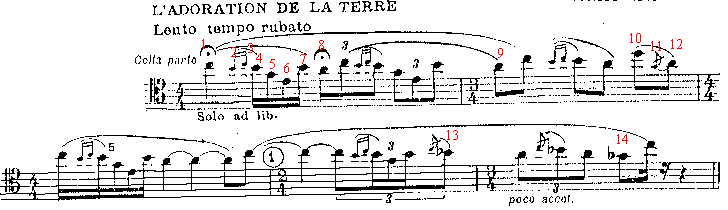F#4 in measure 15 (3 after rehearsal number 3):

```F#4. Note #15:
Eb
/ x x | x x o F```

Grace-note A4 in m. 15 (3 after rehearsal number 3). Same as Cooper p. 288:

```A4. Note #16:
Eb
x x x | o o o F
a c#```

D#4 in m. 15:

```D#4. Note #17:
Eb
o x x | o o o
c#```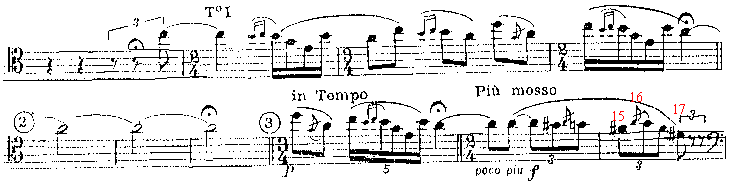Stravinsky, Igor. Rite of Spring, opening C5. Porwit, Timothy. In Apfelstadt, Marc and Klimko, Ronald. Bassoon Performance Practice, Teaching Materials, Techniques and Methods. Idaho: University of Idaho, 1993, p. 60. Start with fingering for C#5 then switch to C5 when reed vibrates.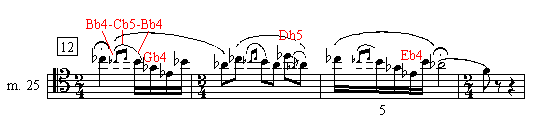Stravinsky, Igor. Rite of Spring, second solo at rehearsal number 12, m. 25, and following. Ewell, Terry B. (20 August 1994). For Bb4-Cb5 grace-notes use Cooper p. 291 or 292 and open L. H. C hole for Cb5. For downward slur to Gb4 use Cooper p. 226.

```Bb4:                    Cb5:
Eb                      Eb
x x x |(x) x o F        x x o | (x) x o F
a c#                    a c#```

Stravinsky, Igor. Rite of Spring, second solo, m. 25, Cb5-Bb4 grace-notes. Houser, Roy. In Klimko, Ronald. Bassoon Performance Practices and Teaching in the United States and Canada. Idaho: University of Idaho, 1974, p. 64.

```Bb4:                   Cb5:
x x x | x x x F       x x o | x x o F
d       Bb            d       Bb```

Stravinsky, Igor. Rite of Spring,mm. 25-28. Moritz, Frederick. "A Dissertation on the Use of Alternate Fingerings for Some of the High Register Notes on the Heckel and Heckel-Type Bassoons." The Double Reed 8/3 (Winter 1985): 28-33. The notes are numbered in the music below. The reader is strongly encouraged to refer to the article, since the fingerings below recur throughout the solos.

Cb5 quarter-note and grace-note in measure 25, at rehearsal number 12 (second solo):

```Cb5. Note #1:
x x o | x o o F
c```

Bb4 grace note and sixteenth note in m. 25:

```Bb4. Note #2:
x x x | x x o F
c       E```

Gb4 in m. 25. Same as Cooper p. 236 except half-hole L. H. D hole:

```Gb4. Note #3:
Eb
o / x | x o o```

Eb4 in m. 25. Same as Cooper p. 191 except add L. H. Eb key:

```Eb4. Note #4:
Eb
x x o | x x x```

Last Bb4 in measure 25:

```Bb4. Note #5:
Eb
x x x | x x o
E```

Ab4 in m. 26 without G# ring. Same as Cooper p. 259 except L. H. no whisper key:

```Ab4. Note #6:
Eb
/ x x | o o x```

Ab4, with G# ring:

```Ab4. Note #6:
Eb
o x x | o o o F```

Db5 in m. 26. Same as Cooper p. 331 except L. H. use only D key; R. H. may add the thumb E key.

```Db5. Note #7:
Eb
x o x | x o x
d        (E)```

Grace-note Gb4 in m. 26. Same as Cooper p. 230 except half-hole L. H. E hole:

```Gb4. Note #8:
Eb
/ x x | x x o
Bb```

Last Ab4 in m. 26. Same as Cooper p. 262 except no whisper key:

```Ab4. Note #9:
Eb
/ x x | x o x
Bb```